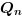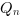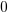Change language to:
English - Français - 日本語 - Português -

See the recommended documentation of this function

Scilab help >> Xcos > palettes > Integer palette > SRFLIPFLOP

# SRFLIPFLOP

SR flip-flop

### Block Screenshot### Description

This block describes the simplest and the most fundamental latch the SR flip flop. The output Q depends of the state of the inputs S and R. The output !Q is the logical negation of Q

• If S (Set) is pulsed high while R is held low, then the Q output is forced high, and stays high when S returns low.

• if R (Reset) is pulsed high while S is held low, then the Q output is forced low, and stays low when R returns low.

• When S and R are low, Q(t) takes the value of the previous output state Q(t-1).

• When S and R are both high, both Q and !Q take the low or high values; the state is unstable. Practically this case is forbidden.

The user can set the initial output state with Initial Value parameter.

This block is almost used as a memory

The truth table of this block is:HoldResetSetForbidden state

where `U` stands for "Unknown".

### Data types

The block supports the following types :

• Inputs:

• R: scalar. Scilab's int8 data type only.

• S: scalar. Scilab's int8 data type only.

A positive input is considered as logical 1, a negative or a null input as logical 0.

• Outputs: scalar. Scilab's int8 data type.

### Dialog box• Initial Value

Initial Value of the state Q. It must be int8 data type.

Properties : Type 'vec' of size 1.

### Default properties

• always active: no

• direct-feedthrough: yes

• zero-crossing: no

• mode: no

• regular inputs:

- port 1 : size [1,1] / type 5

- port 2 : size [1,1] / type 5

• regular outputs:

- port 1 : size [1,1] / type 5

- port 2 : size [1,1] / type 5

• number/sizes of activation inputs: 0

• number/sizes of activation outputs: 0

• continuous-time state: no

• discrete-time state: no

• object discrete-time state: no

• name of computational function: csuper

### Example

The following example presents a typical anti-bouncing application of the SR flipflop. The output graph shows the memory effect of the flipflop. Open this example in Xcos